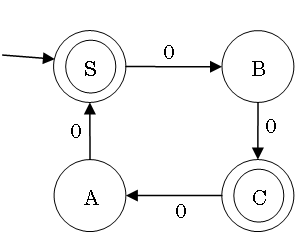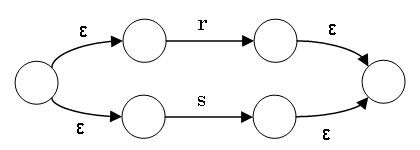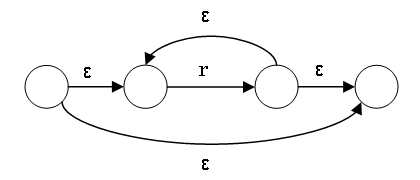# Regular Expressions

(正規表現)

## Language Theory and Compilers

http://www.sw.it.aoyama.ac.jp/2019/Compiler/lecture4.html

### Martin J. Dürst© 2005-19 Martin J. Dürst 青山学院大学

# Today's Schedule

• Last week's homework, leftovers
• Minimization of DFAs
• Regular Expressions
• Formal definition
• Conversion to an NFA
• Conversion from an FSA
• Regular expressions in practice

# Today's Outlook

Summary from last time:

• Finite state automata (FSA): deterministic finite automata (DFA) and non-deterministic finite automata (NFA)
• Regular grammar: left linear grammar and right linear grammar
• All these have the same power, generating/recognizing regular languages.

Callenge: Regular languages can be represented by state transition diagrams/tables of NFAs/DFAs, or with regular grammars, but a more compact representation is desirable.

There is a very powerful way to represent regular languages, called regular expressions

# Minimization of DFAs

To create the smallest DFA equivalent to a given DFA:

Overall idea: work backwards

1. Separate states into two sets, accepting states and non-accepting states
2. For each state, check which other states are reached for each input symbol
3. Partition each set of states into sets that can reach the same set with the same input symobls
4. Repeat 2. and 3. until there is no further change

Purpose of minimization:

• Efficient (minimum memory) implementation
• Deciding whether two FSAs are equivalent
(they are equivalent if their minimized DFAs are isomorphic)

# Example of DFA Minimization# Efficient Implementation of a DFA

```State   next_state[state_count][symbol_count]; /* state transition table */
Boolean final_state[state_count];              /* final state? */
State   current_state = start_state;
Symbol  next_symbol;

while ((next_symbol=getchar()) != EOF &&       /* end of input */
current_state != no_state)            /* dead end */
current_state = next_state[current_state][next_symbol];
if (final_state[current_state])
printf("Input accepted!");
else
printf("Input not accepted!");```

# Application of Regular Expressions

Problem 04C1 of Computer Practice I: Convert `&amp;`, `&quot;`, `&apos;`, `&lt;` and `&gt;` in the input to `&`, `"`, `'`, `<`, and `>`, respectively.

One way to write this in Ruby:

`gsub /&quot;/, '"'gsub /&apos;/, "'"gsub /&lt;/,   '<'gsub /&gt;/,   '>'gsub /&amp;/,  '&'`

`gsub` replaces all occurrences of a give pattern in a string

`//` are the delimiters for regular expressions (in Ruby, Perl, JavaScript,...)

Regular expressions match some input.

# Regular Expressions

• Expression to denote a set of patterns or words (i.e. a language)
• Very compact
• Widely used, very useful
• Two main variants:
• Theoretical
• Practical

# Regular Expressions

• Expression to denote a set of patterns or words (i.e. a language)
• Very compact
• Widely used, very useful
• Two main variants:
• Theoretical
• Practical

# (Theoretical) Regular Expression: Basic Syntax

• `a`: {a} (a single symbol denotes itself)
• `abc`: {abc} (concatenation, single word)
• `a*`: {ε, a, aa, aaa,...} (Kleene closure)
• `a|b`: {a, b} (alternative)
• Combinations:
• `ab|c*|d`: {ab, ε, c, cc, ccc,..., d}
• `a(b|c)*d`: {ad, abd, acd, abbd, abcd, acbd, accd,...}

# More Examples of Regular Expressions

• Combinations:
• `ab|c*|d`: {ab, ε, c, cc, ccc,..., d}
• `a(b|c)*d`: {ad, abd, acd, abbd, abcd, acbd, accd,...}
• Number of symbols:
• Even: `(aa)*`
• Odd: `a(aa)*` or `(aa)*a`
• Reminder is 2 when divided by 3: `aa(aaa)*`
• A specific symbol sequence at the start of a word: `abc(a|b|c)*`
• A specific symbol sequence at the end of a word: `(a|b|c)*abc`
• A specific symbol sequence in the middle of a word: `(a|b|c)*abc(a|b|c)*`

# Why Regular Expressions?

• It is possible to use a regular grammar to define a regular language
• A grammar has multiple rewriting rules, and is difficult to understand
• A single regular expression can represent a whole regular language.
This regular expression is easy to write and read because it is short.

# Notation of Regular Expressions

• Only characters themselves, concatenation, alternative, and repetition are represented
• "Usual" characters represent themselves
• A small set of characters has a special role (meta-characters: `|`, `*`, `(`, `)`, ε)
• Meta-characters may have to be escaped

# Formal Definition of Regular Expressions

Theoretical Regular Expressions over Alphabet Σ
Priority Regular Expression Condition Defined Language Notes

ε, a a ∈ Σ {ε} or {a} literals
very high (r) r is a regular expression L((r)) = L(r) grouping
high r* r is a regular expression L(r*) = (L(r))* Kleene closure
low rs r, s are regular expressions L(rs) = L(r)L(s) concatenation
very low r|s r, s are regular expressions L(r|s) = L(r) ∪ L(s) set union

L(r) is the language defined by regular expression r

# Caution: Priority

Make sure you understand the difference between the following pairs of regular expressions:

• abc* vs. (abc)*
• a|b|c* vs. (a|b|c)*
• ab|c vs. a(b|c)

# Grammar for Regular Expressions

• Regular expressions also form a language
(set of all regular expressions)
• Grammar: R → ε, R →a, R →b,..., RR|R, RRR, RR*, R →(R)
• This is not a regular language, but a context-free language
• The alphabet of a regular expression is the alphabet of the target language (e.g. a, b,...) and the meta-characters (ε, |, *, (, ))

# Regular Expression to NFA

• Construct NFA bottom-up, starting with smallest subexpressions
• Each subexpression is converted to an NFA
• Each subexpression has one start state and one accepting state
• When combining subexpressions, connect start states and accepting states to form a larger NFA (see next two slides)
• During construction:
• Start state is on the left (no need for incomming arrow)
• Accepting state is on the right (no need for double circle)
• When finished, do not forget to add incomming arrow for start state and double circle for accepting state

# Regular Expression to NFA: Symbols, Alternatives

The NFA for a symbol a has a start state and an accepting state, connected with a single arrow labeled a (same for ε)

The NFA for r|s is constructed from the NFAs for r and s as follows:The additional ε connections are necessary to clearly commit to either r or s.

# Regular Expression to NFA: Concatenation, Repetition

The NFA for the regular expression rs connects the accepting state of r with the start state s through an ε transition. The overall start state is the start state of r; the overall accepting state is the accepting state of s.

The NFA for r* is constructed as follows:# Example of Conversion

Regular expression: `a|b*c`

In some cases, some of the ε transitions may be eliminated, or the NFA may otherwise be simplified.

# From FSA to Regular Expression

Algorithmic conversion is possible, but complicated

General procedure:

1. Create regular expressions for getting from state A to state B directly for all pairs of states
2. Select a single state, and create all regular expressions that pass through this intermediate state
3. Repeat step 2., increasing the number of intermediate states
4. Simplify intermediate regular expressions as much as possible (they can get quite complex)

When understanding what language the FSA accepts, it is often easy for humans to create a regular expression for this language.

# Applications of Regular Expressions

• Many different patterns can be expressed in a compact form
• Clear connection between theory and applications
• Built-in to many programming languages (Ruby, Javascript, Perl, Python,...)
• Available as libraries in other programming languages (Java, C#, C,...)
• Usable in many tools (e.g. plain text editors)
• Caution: Theoretical regular expressions and practical regular expressions differ in many ways

# Practical Regular Expressions: Notational Differences

Practical regular expressions have many additional functions and shortcut notations
(the corresponding theoretical regular expressions or simpler constructs are given in parentheses)

• `.`: a single arbitrary character (`a|b|c|`...)
• `[acdfh]`: character class: select a single character (`(a|c|d|f|h)`)
• `[b-f]`: shortcut for continuous range in character class (`(b|c|d|e|f)`)
• r`+`: one or more occurrences of r (rr`*`)
• r`?`: r or nothing (r|ε, ε cannot be used in practical regular expressions
• r`{`m`,`n`}`: between m and n repetitions of r (r...rr`?`...r`?`)
• `\*`,...: `\` escapes meta-characters
• Meta-characters: `|*+?()[]{}.\^\$`

# Practical Regular Expressions: Usage Differences

• Theory: match a full word; practice: match part of a string
• `^`/`\$` match the start/end of a string or line
• The result of the match is not just yes/no, but includes the position of the match, the substring matched, the substrings before/after the match,...
• If there are multiple possible matches, the leftmost, longest match is choosen
(leftmost is more important than longest)
• Parts of a string matching parts of a regular expression in parentheses can be assigned to variables
• Partial matches can be reused inside the regular expression

# Use of Practical Regular Expressions

• Text/document search
• String replacements (single or multiple)
• Cutting strings apart

# Notes on Practical Regular Expressions

• Most regular expression engines are more powerful than DFA/NFA/regular languages
• Most regular expression engines use backtracking
• Some regular expressions may be very slow on some input
Example: String an, regular expression a?nan (n=3: string: aaa, regular expression: a?a?a?aaa, really slow starting at , n~25)
• For further analysis, see e.g. https://regex101.com/

# Theoretical vs. Practical Regular Expressions

Theoretical Practical `* | ( )` `|*+?()[]{}.\^\$` yes no no yes no yes no yes full word part of a string

# Summary of this Lecture

• Regular expressions, regular grammars, and finites state automata all
have the same power to generate/accept regular languages
• Regular expressions are a very compact representation
• DFAs are a very efficient way to implement recognition
• These are very useful for lexical analysis
• However, creating a DFA by hand from a regular expression is tedious
• However, because the number of states is finite, there are languages that cannot be expressed, e.g. languages with corresponding pairs of parentheses

# Homework

Deadline: May 21, 2018 (Thuesday!), 19:00

Where to submit: Box in front of room O-529 (building O, 5th floor)

Format: A4 single page (using both sides is okay; NO cover page), easily readable handwriting (NO printouts), name (kanji and kana) and student number at the top right

1. Construct the state transition diagram for the NFA corresponding to the following grammar
S → εA | bB | cB | cC, A → bC | aD | a | cS, B → aD | aC | bB | a, C →εA | aD | a
(Caution: In right linear grammars, ε is not allowed except in the rule S → ε)
(Hint: Create a new accepting state F)
2. Convert the following transition table to a right linear grammar
 0 1 →T G H *G K L *H M K *K K K *L M K M L -
3. Construct the state transition diagram for the regular expression `ab|c*d`
(write down both the result of the procedure explained during this lecture (with all ε transitions) as well as a version that is as simple as possible)
4. Bring your notebook PC (with `flex`, `bison`, `gcc`, `make`, `diff`, and `m4` installed and usable)

# Glossary

regular expression

minimization

partition

isomorphic

delimiter

alternative

repetition

meta-character
メタ文字
priority

theoretical regular expressions

practical regular expressions

notation(al)

arbitrary

leftmost
できるだけ左